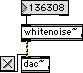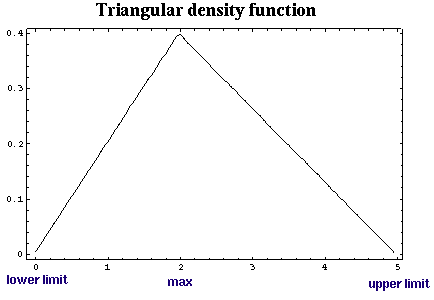# Digital Music Programming II: whitenoise~

This lab demonstrates how send audio signals out of an MSP object as well as generate whitenoise.

Whitenoise by definition contains all frequencies at a constant amplitude. To generate whitenoise is very simple in theory: each sample of whitenoise is a uniformly random value in the range from [-1.0 .. 1.0).

The actual creation of the whitenoise is done in the program below with the following line of code:

```      output[i] = randfloat() * 2.0 - 1.0;
```
where randfloat() returns a random number between 0.0 and almost up to (but not quite) 1.0. The random number between 0 and 1 is multiplied by 2 to get it in a range from 0 to 2, then 1 is subtracted from the number in the range from 0 to 2 to generate a number in the range from -1 to 1 which is the correct range for audio output in Max/MSP.

To get a random number in C which is in the range from [0.0 to 1.0), you have to do this:

```   (float)rand()/RAND_MAX;
```
Where rand() generates a random integer from 0 up to some number, RAND_MAX (or RAND_MAX-1 perhaps). To get a random number between 0.0 and 1.0, divide by RAND_MAX. You must be careful not to use integer division by casting the rand() output to a float before dividing by RAND_MAX; otherwise, you will end up with always getting the value of 0.0 as a result due to integer division:
```   rand()/RAND_MAX;       // always 0
```

RAND_MAX is defined to be the highest number the rand() function can output. This value is either the largest 16-bit signed number or the largest 32-bit signed number. It depends on what compiler you are using.

The rand() function generates a pseudo-random sequence of numbers. This sequence is not actually random, and can be generated exactly given the same starting condition. A seed can be used to control the starting condition. The srand() function can be used to set the starting condtion for the rand() function. srand() takes a long integer as input an starts the sequence of random numbers based on that starting value.

The function time(NULL) returns the current time in seconds since Jan 1, 1970, (0:00 UTC). So every time you start the Max/MSP program, the whitenoise will be based on a completely different pseudo-random sequence unless you seed it explictly with a different value on input 1 to the object.

On a POSIX, computer, I would recommend using the drand48() function in place of (float)rand()/RAND_MAX because it is much more closer to a uniform distribution of random numbers:

```   float randfloat(void) {
return (float)drand48();
}
```
drand48() generates a random double float in the range from 0.0 to 1.0. You would use srand48() to seed the drand48() function just like the srand() function.

 Source Code Example Usage whitenoise.c``` #include "ext.h" #include "z_dsp.h" #include /* included for rand() function */ #include /* included for time() function */ typedef struct { t_pxobject msp_data; } MyObject; void* object_data; void main (void); void* create_object (void); void InputSeed (MyObject* mo, long value); void MessageDSP (MyObject* mo, t_signal** signal, short* count); t_int* Perform (t_int *parameters); float randfloat (void); void main(void) { setup((t_messlist**)&object_data, (method)create_object, (method)dsp_free, (short)sizeof(MyObject), NULL, A_NOTHING); addint((method)InputSeed); addmess((method)MessageDSP, "dsp", A_CANT, A_NOTHING); dsp_initclass(); } void* create_object(void) { MyObject *mo = (MyObject*)newobject(object_data); dsp_setup((t_pxobject*)mo, 0); outlet_new((t_pxobject*)mo, "signal"); InputSeed(mo, time(NULL)); return mo; } void InputSeed(MyObject *mo, long value) { #pragma unused(mo) srand(value); } void MessageDSP(MyObject* mo, t_signal** signal, short* count) { #pragma unused(mo) #pragma unused(count) dsp_add(Perform, 3, 3, signal->s_vec, signal->s_n); } t_int* Perform(t_int *parameters) { long pcount = (long) (parameters); t_float *output = (t_float*) (parameters); long count = (int) (parameters); long i; for (i=0; i

# Exercises

1. Compile and listen to the whitenoise~ object.

2. Increase the amplitude of the whitenoise object by using the *~ object. As you decrease the volume of the whitenoise to 0, how does the sound qualilty change? As you increase the volume of the whitenoise to 100 (or whatever), how does the sound quality change? What is happening in the second case?

3. Make your own noise generate that generate noise other than whitenoise. Try another distribution besides uniform distribution to generate the noise. For example, try the following triangular distribution:Three control parameters:

1. lower limit (use -1.0, for example)
2. upper limit (use +1.0, for example)
3. maximum (choose 0.0 for minimizing DC component, for example)

For the simple case with -1 as the lower limit, 0 as the max and, +1 as the upperlimit, you can use this function in place of the floatrand() function in the program above.

```
float trianglerand(void) {
return floatrand() + floatrand() / 2.0;
}
```

Here is a function to generate a triangular distribution in the generalized case where trianglerand() would replace the code floatrand() * 2.0 - 1.0:

```
float trianglerand(float lower, float upper, float max) {
float norm = floatrand()+floatrand();
float output;

// scale each side of the triangle to the requested range:
if (norm < 1.0) {
output = (norm - 1.0) * lower + max;
} else {
output = (norm - 1.0) * upper + max;
}

// normalize the sides of the triangle so that the probability
// densities matchup at the maximum:
if (lower == upper) {
return output;
} else if (output < max && lower < upper) {
if (floatrand() < (float)lower/upper) {
return output;
} else {
return trianglerand();
}
} else if (output > max && upper < lower) {
if (floatrand() < (float)upper/lower) {
return output;
} else {
return trianglerand();
}
}

return output;
}

```
```

```